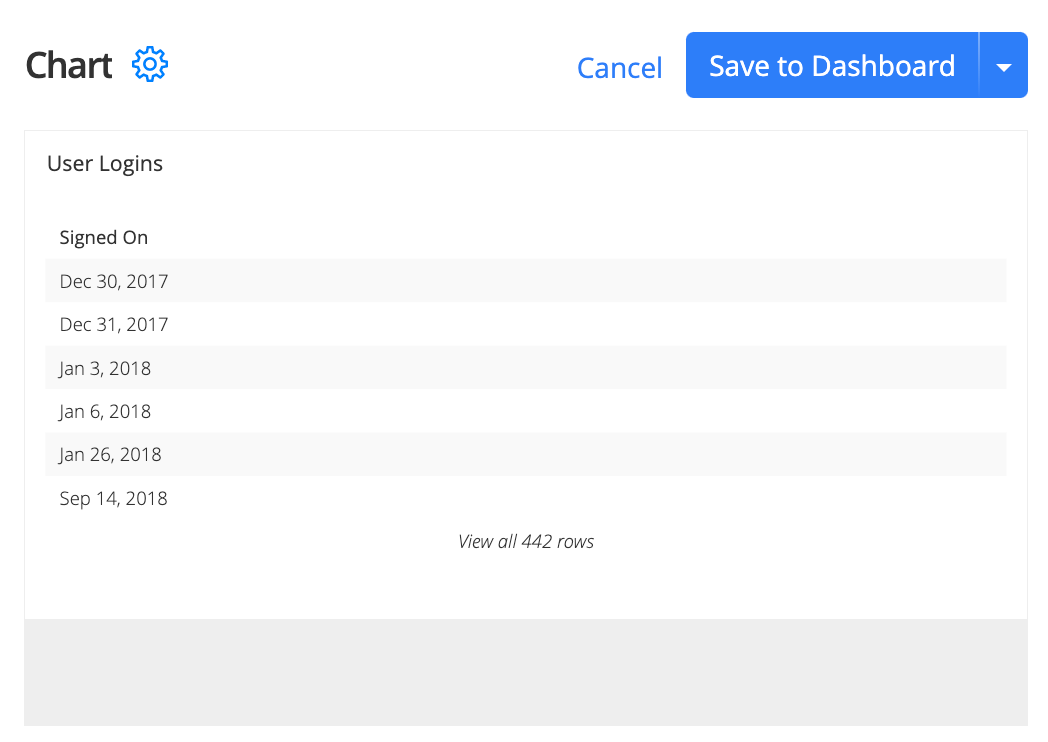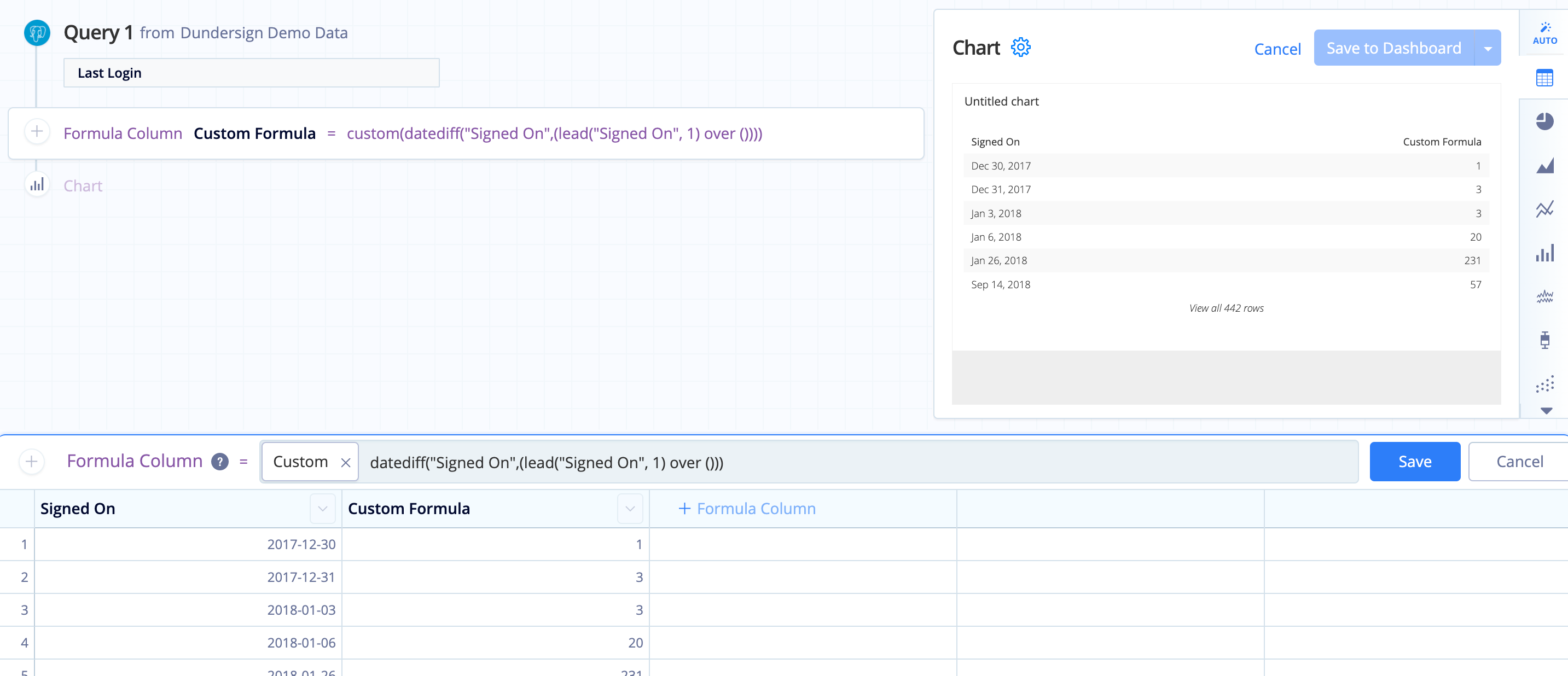# Find the date difference between rows in Visual SQL

When wanting to calculate the difference in time between two date columns, the Date difference formula option in the Formula Column or Apply Formula Actions can be used.

In the event you only have one date column but would like to calculate the difference between each row, you can instead use the `datediff()` function in a Custom formula.

## Using the lag() function

An easy way to do this is to use the lag() function—which we’ve conveniently turned into a Guided Formula you can use—in your Formula Column or Apply Formula Action to create a start column to use as part of your date calculation.

In the example below, we’ll calculate the number of days between user login times.1. First, query the column containing the login dates and run the query. For this example, we’ll use the Last Login column from the Users table of our Dundersign Demo Data data source. We rename the column to Signed On.
2. Add a Formula Column, which will act as the start date to calculate the date difference. We’ll then select the Lag Guided Formula and set a Row Offset of `1`. Click Save to save the Action.

3. After creating the offset column, we’ll want to create another column to calculate the number of days between login dates. To do this, we add another Formula Column and select the Date Difference Guided Formula. Then, select the start and end columns as well as the unit of time. For this example, the start date column is Lag, the end date column is Signed On, and the unit of time is `day`. Click Save to save the Action.

Instead of the Guided Formula option, you can also use the `datediff()` built-in formula below as part of a Custom formula, replacing the `Start Date Column` and `End Date Column` with the names of your columns and `unit` with the unit of time you’d like to calculate (the `unit` argument is optional):

``````datediff("Start Date Column","End Date Column",'unit')
``````

Note: If you choose not to specify a unit in the formula, it will default to calculate days.

Another option is to skip adding an additional lag column and use the lead() window function in place of the end column in your `datediff()` formula. This function acts similarly to the `lag()` function we used above and will populate with the end date automatically.

To do this, add a Formula Column, choose Custom formula for the formula type, then use the formula below, replacing `Date Column` in the formula with the name of your date column (the `order by` clause is optional):

``````datediff("Date Column",(lead("Date Column", 1) over (order by "Date Column")))
``````

where the ORDER BY clause in the OVER clause is optional; you could simply use `over ()` to use the current order of rows in the selected column.

Here, we simply wanted to keep the current order of date rows; therefore, we omitted the ORDER BY clause from the formula as follows:

``````datediff("Signed On",(lead("Signed On", 1) over ()))
``````You now have the number of days between login dates and can perform additional calculations as needed.